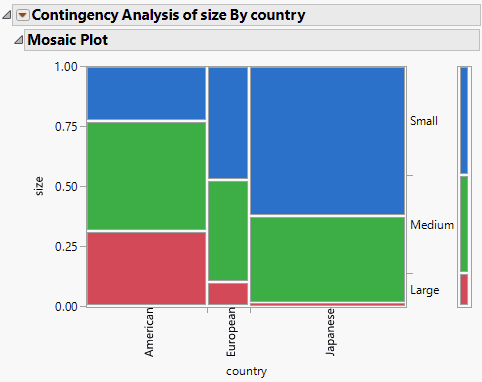Publication date: 11/29/2021

# Mosaic Plot

The mosaic plot is a graphical representation of the two-way frequency table or Contingency Table. A mosaic plot is divided into rectangles; the vertical length of each rectangle is proportional to the proportions of the Y variable in each level of the X variable. The mosaic plot was introduced by Hartigan and Kleiner (1981) and refined by Friendly (1994).

To produce the plot shown in Figure 7.5, follow the instructions in Example of Contingency Analysis.

Figure 7.5 Example of a Mosaic PlotNote the following about the mosaic plot:

The proportions on the horizontal axis represent the number of observations for each level of the X variable, which is country.

The proportions on the vertical axis at right represent the overall proportions of Small, Medium, and Large cars for the combined levels (American, European, and Japanese).

The scale of the vertical axis at left shows the response probability. The whole axis is equivalent to a probability of one (representing the total sample).

Clicking on a rectangle in the mosaic plot highlights the selection and highlights the corresponding data in the associated data table.

Replace variables in the mosaic plot by dragging and dropping a variable, in one of two ways: swap existing variables by dragging and dropping a variable from one axis to the other axis; or, click a variable in the Columns panel of the associated data table and drag it onto an axis.